# Pascal's Triangle facts for kids

Kids Encyclopedia Facts
1
1   1
1   2   1
1   3   3   1
1   4   6   4   1
1   5  10  10   5   1

The first six rows of Pascal's triangle

Pascal's Triangle is a mathematical triangular array. It is named after French mathematician Blaise Pascal, but it was used in China 3 centuries before his time.

Pascal's triangle can be made as follows. On the first row, write only the number 1. Then, to get the numbers of following rows, add the number that can be seen above and to the left (if any) and the number above and to the right (if any) to find the new value. For example, the numbers 1 and 3 in the fourth row are added to make 4 in the fifth row.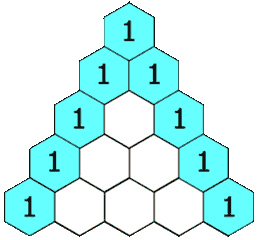Each number in the triangle is the sum of the two directly above it.Yang Hui (Pascal's) triangle, as depicted by the Chinese using rod numerals.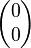$\begin{pmatrix} 0 \\ 0 \end{pmatrix}$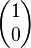$\begin{pmatrix} 1 \\ 0 \end{pmatrix}$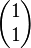$\begin{pmatrix} 1 \\ 1 \end{pmatrix}$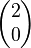$\begin{pmatrix} 2 \\ 0 \end{pmatrix}$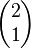$\begin{pmatrix} 2 \\ 1 \end{pmatrix}$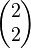$\begin{pmatrix} 2 \\ 2 \end{pmatrix}$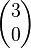$\begin{pmatrix} 3 \\ 0 \end{pmatrix}$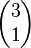$\begin{pmatrix} 3 \\ 1 \end{pmatrix}$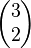$\begin{pmatrix} 3 \\ 2 \end{pmatrix}$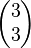$\begin{pmatrix} 3 \\ 3 \end{pmatrix}$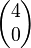$\begin{pmatrix} 4 \\ 0 \end{pmatrix}$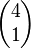$\begin{pmatrix} 4 \\ 1 \end{pmatrix}$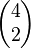$\begin{pmatrix} 4 \\ 2 \end{pmatrix}$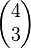$\begin{pmatrix} 4 \\ 3 \end{pmatrix}$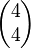$\begin{pmatrix} 4 \\ 4 \end{pmatrix}$

## Uses of Pascal triangle

The triangle has many uses in probability. It can also be used in binomial expansions. For example

(x + 1)2 = 1x2 + 2x + 12.

Notice the coefficients are the third row of Pascal's triangle: 1, 2, 1. In general, when a binomial is raised to a positive integer power we have:

(x + y)n = a0xn + a1xn−1y + a2xn−2y2 + … + an−1xyn−1 + anyn,

where the coefficients ai in this expansion are precisely the numbers on row n + 1 of Pascal's triangle; in other words,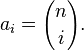$a_i = {n \choose i}.$

## Pascal's rule

Formally speaking, the way of making the Pascal's Triangle uses Pascal's rule, which states that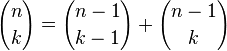${n \choose k} = {n-1 \choose k-1} + {n-1 \choose k}$

for non-negative integers n and k where nk and with the initial condition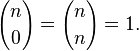${n \choose 0} = {n \choose n} = 1.$

## Complicated structures based on Pascal's triangle

Pascal's triangle generalizes readily into higher dimensions. The three-dimensional version is called Pascal's pyramid or Pascal's tetrahedron. A higher-dimensional analogue is generically called a "Pascal's simplex"*. See also pyramid, tetrahedron, and simplex.

## Properties of Pascal's Triangle

The first and last number in each row is always 1.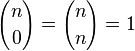${n \choose 0} = {n \choose n}=1$

The second number in each row is the row number. In a binomial expansion, this second number is equal to the power. (This makes the 'first' row, 1, actually the zero row).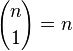${n \choose 1} = n$

The rows always read the same forward and backward.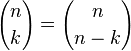${n \choose k} = {n \choose n-k}$

The sum of the numbers in each row is double the sum of the numbers in the row before. The sum of each row is 2n.

The zero row, 1, has a sum of 1.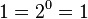$1=2^0=1$
The first row, 1,1 has a sum of 2.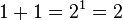$1+1=2^1=2$
The second row, 1,2,1 has a sum of 4.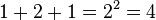$1+2+1=2^2=4$
The third row, (1,3,3,1) has a sum of 8.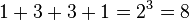$1+3+3+1=2^3=8$
The sum of the nth row is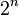$2^n$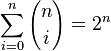$\sum_{i=0}^n {n \choose i} = 2^n$

## Images for kidsPascal's Triangle Facts for Kids. Kiddle Encyclopedia.